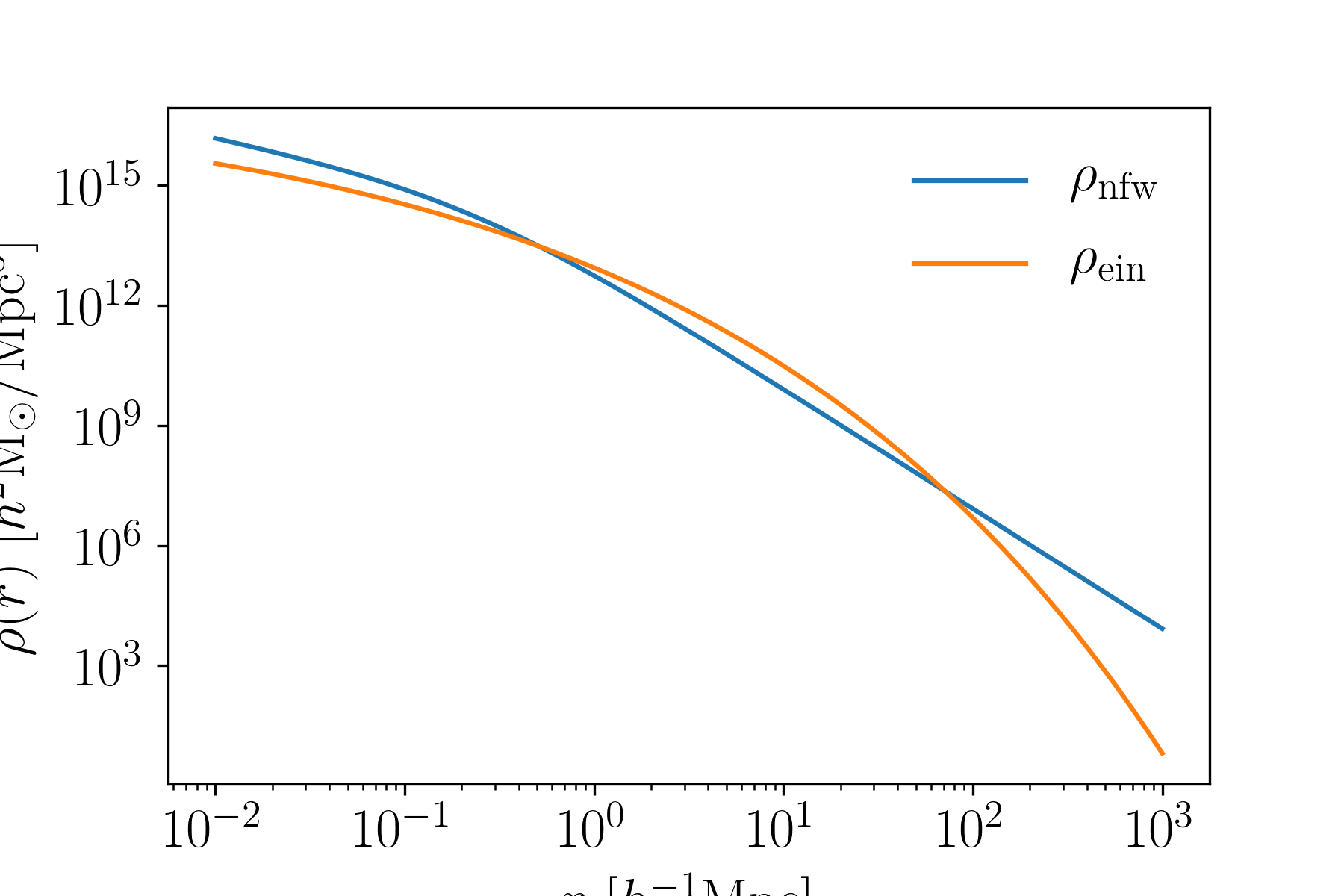# Density Profiles¶

Most cluster observables are closely related to their density profiles. This repository contains routines for calcalating the NFW and Einasto profiles.

## NFW Profile¶

The NFW profile (arxiv) is a 3D density profile given by:

$\rho_{\rm nfw}(r) = \frac{\Omega_m\rho_{\rm crit}\delta_c}{\left(\frac{r}{r_s}\right)\left(1+\frac{r}{r_s}\right)^2}.$

The free parameters are the cluster mass $$M_\Delta$$ and concentration $$c_\Delta = r_\Delta/r_s$$. In this module we choose to define the density with respect to the matter background density $$\Omega_m\rho_{\rm crit}$$. The scale radius $$r_s$$ is given in $$h^{-1}{\rm Mpc}$$, however the code uses concentration $$c_\Delta$$ as an argument instead. The normalization $$\delta_c$$ is calculated internally and depends only on the concentration. As written, because of the choice of units the only cosmological parameter that needs to be passed in is $$\Omega_m$$.

Note

The density profiles can use $$\Delta\neq 200$$.

To use this, you would do:

from cluster_toolkit import density
import numpy as np
radii = np.logspace(-2, 3, 100) #Mpc/h comoving
mass = 1e14 #Msun/h
concentration = 5 #arbitrary
Omega_m = 0.3
rho_nfw = density.rho_nfw_at_r(radii, mass, concentration, Omega_m)


## Einasto Profile¶

The Einasto profile is a 3D density profile given by:

$\rho_{\rm ein}(r) = \rho_s\exp\left(-\frac{2}{\alpha}\left(\frac{r}{r_s}\right)^\alpha\right)$

In this model, the free parameters are the scale radius $$r_s$$, $$\alpha$$, and the cluster mass $$M_\Delta$$. The scale density $$\rho_s$$ is calculated internally, or can be passed in instead of mass. To use this, you would do:

from cluster_toolkit import density
import numpy as np
radii = np.logspace(-2, 3, 100) #Mpc/h comoving
mass = 1e14 #Msun/h
r_scale = 1.0 #Mpc/h comoving scale radius
alpha = 0.19 #arbitrary; a typical value
Omega_m = 0.3
rho_ein = density.rho_einasto_at_r(radii, mass, r_scale, alpha, Omega_m)


We can see the difference between these two profiles here: